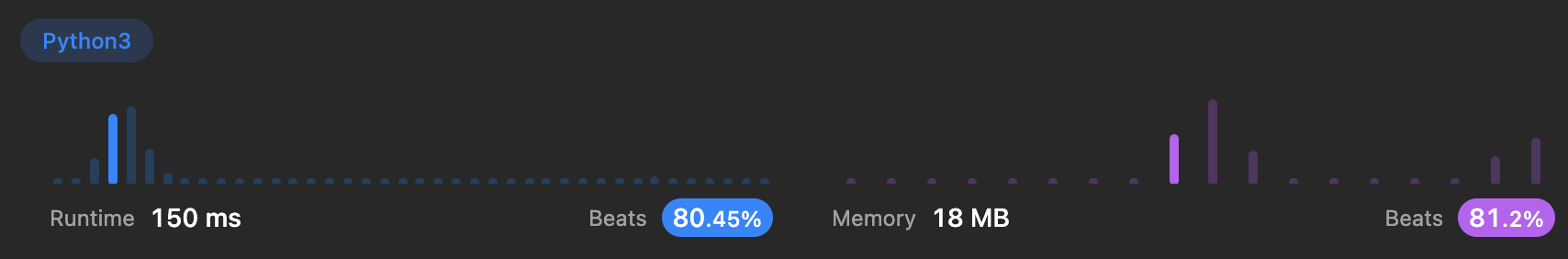Algorithm Puzzles everyday every week sometimes: Merge Intervals

## Puzzle

Puzzle from leetcode:

Given an array of intervals where intervals[i] = [starti, endi], merge all overlapping intervals, and return an array of the non-overlapping intervals that cover all the intervals in the input.

## Solution

Sort input list, then find overlapsT.C. should be O(N * logN)

Sort takes O(N * logN)